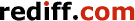Print this article

# Has one of mathematics's toughest problems been solved?

October 20, 2018 11:18 IST

Mathematical problems can sometimes turn out to have real-world applications.
This is true for the Riemann Hypothesis.
Crypto-currency investors will heave a sigh of relief if the problem is unsolved since a proof could render their expertise obsolete, explains Devangshu Datta.
Illustration: Uttam Ghosh/Rediff.comA 90-year-old genius, Sir Michael Atiyah, recently claimed to have proved one of the most esoteric of mathematical conjectures, the Riemann Hypothesis (RH).

His 'solution' is probably wrong, but it has not yet been definitively rejected by peer review.

Cryptographers and crypto-currency investors must have heaved a sigh of relief at the problem remaining unsolved since a proof could render their expertise obsolete.

Mathematical problems that start as abstract exercises can sometimes turn out to have real-world applications. This is true for the RH.

There's a \$1 million Millennium Prize from the Clay Mathematics Institute for finding a valid proof (or disproof) of the RH. If Atiyah is wrong, that prize is still up for grabs.

In 1859, German mathematician Bernhard Riemann (1826-1866) had an insight into the way numbers are distributed. But he couldn't prove it.

Riemann was working with complex numbers (numbers with an imaginary component, 'i', which is defined as the square root of minus 1). While calculating a series known as the Zeta Function, he noticed a property that he conjectured to be true for all numbers.

If he was correct, the Zeta Function tells us a lot about primes -- numbers that can only be divided by themselves and one.

The RH is true till the 100 billionth test. New theorems have been generated by conditionally assuming Riemann was correct. However, there is an infinity of numbers (actually, many infinities) and simply because Riemann was correct 100 billion times doesn't mean the hypothesis is true.

Any proof/disproof could crack the foundations of modern cryptography.

It could also lead to insights into quantum theory, since there seems to be a strong correspondence between energy levels in quantum physics and the pure maths of the RH.

Our civilisation depends on the secure encrypted exchanges, and processing, of digital data.

That encryption can be of varying strengths. But although encryption may vary in strength, the basic principles are similar for most modern encryption methods.

A proof of the RH may include a magic formula that breaks into common encryption systems.

A four-digit ATM PIN is one of 10,000 combinations that could be guessed in a jiffy by a fast computer.

A bank password of 15 mixed characters is magnitudes stronger.

Military encryption standards are very strong and decoding a military message could, in theory, take very fast computers millions of years.

Computers multiply and divide by simple addition and subtraction.

Commonly, encryption is built around the fact that it is far easier to multiply than to divide.

For example, 101 can be multiplied by 409 (both primes) by simply adding 409 to itself 101 times to reach 41,309.

Dividing 41,309 involves dividing it first by 2, then 3, then 5, 7, and so on, until you discover 101 is a factor.

A computer will subtract 2 from 41,309, all of 20,655 times before rejecting 2 as a factor.

Then it will subtract 3, 13,769 times before rejecting 3 as a factor, and so on.

For example, 'ABCD' could be converted into '1234' (substituting numbers for letters) multiplied by 41,309.

To decode this simple code, you must divide '50,975,306' by 41,309. Try doing this without knowing the factors!

There are many tricks to speed up such computations. But factorisation is really hard for large primes.

Digital encryption systems are based on using 30-digit, or longer, prime numbers.

Crypto-currencies like bitcoin also rely on primes for encryption.

TGreek mathematician Euclid proved there are an infinity of primes. There is no easy way to find a prime, or to work out how many primes are in a given range.

here are 1,229 primes between 1 and 10,000, for example. Proving the RH would, almost certainly, involve developing methods for predicting prime distributions.

That would make it much easier to find primes, and break codes.IMAGE: Has Sir Michael Atiyah proved the Riemann Hypothesis? Photograph: Kind courtesy newton.ac.uk

Atiyah has, in his time, received both the Abel Prize and the Fields Medal -- that's the equivalent of two Nobel Prizes for mathematicians. But mathematicians do their best work before turning 40 and the odds are against the nonagenarian's proof being valid.

His explanatory lecture at Heidelberg was charming, but unsatisfying.

Atiyah was working on a physics problem of calculating the Fine-Structure Constant (this measures electromagnetic attraction between particles) when he thought up his 'proof'. (Physicists say his Fine Structure Constant calculation is also flawed.)

He used an approach of reductio ad absurdum by initially assuming the RH was wrong. If it is wrong, certain things must follow. Since those things don't follow, the RH must be correct.

This approach is generally treated with suspicion by mathematicians and the initial consensus is, the proof isn't convincing.

The angle of approach, coming from physics to pure maths, suggests there may be some route to a proof from the real world.

In the meantime, bitcoin, ethereum, and so on, can trade without fear that somebody will suddenly hack the mining process.

Devangshu Datta